## RS Aggarwal Class 8 Solutions Chapter 1 Rational Numbers Ex 1D

These Solutions are part of RS Aggarwal Solutions Class 8. Here we have given RS Aggarwal Solutions Class 8 Chapter 1 Rational Numbers Ex 1D.

Other Exercises

Question 1.
Solution: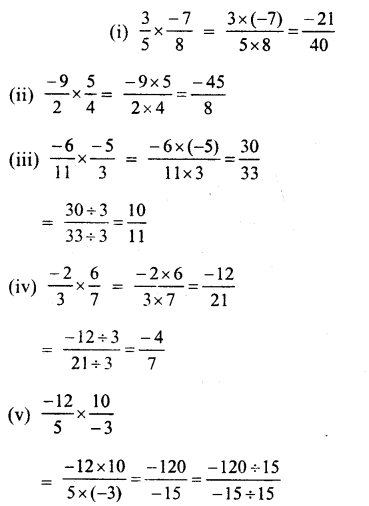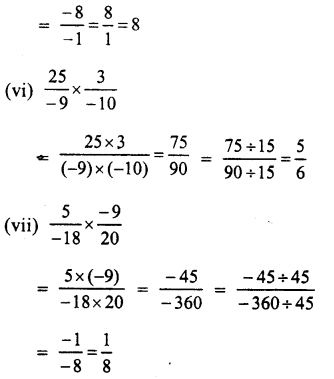Question 2.
Solution: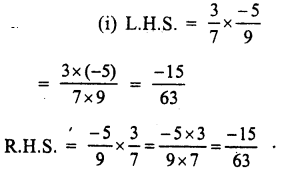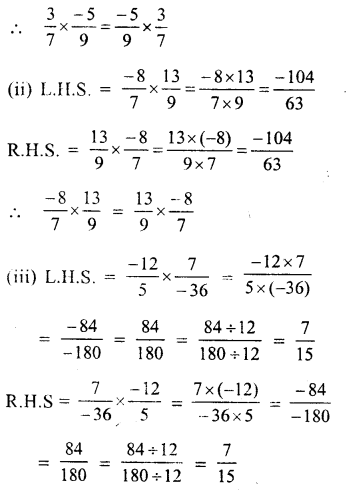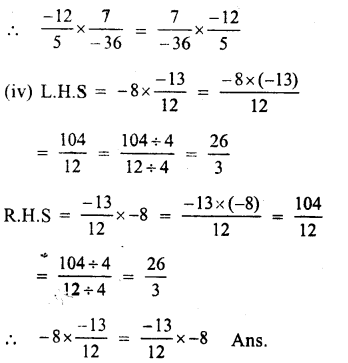Question 3.
Solution: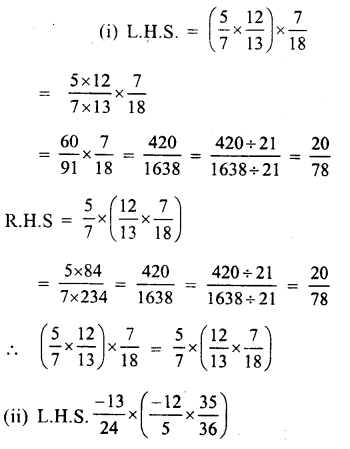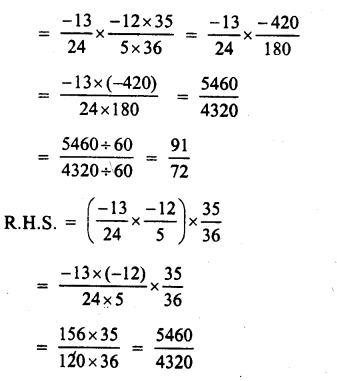Question 4.
Solution: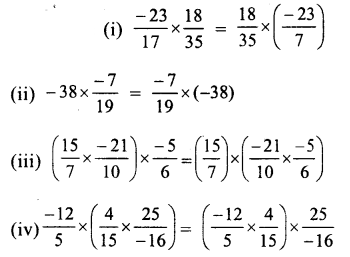Question 5.
Solution: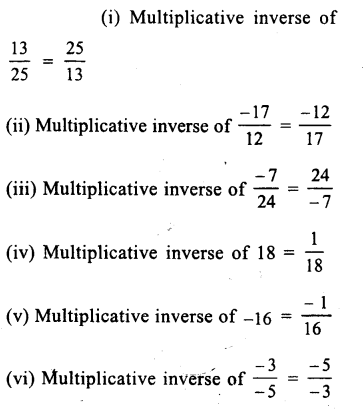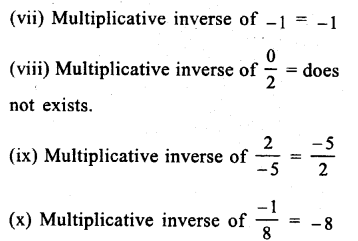Question 6.
Solution: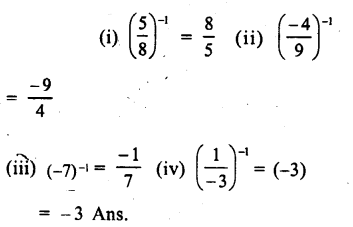Question 7.
Solution: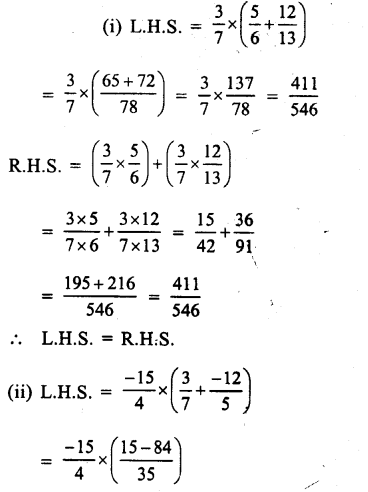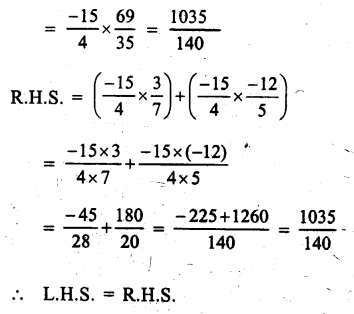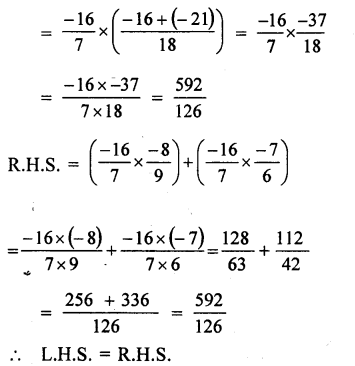Question 8.
Solution: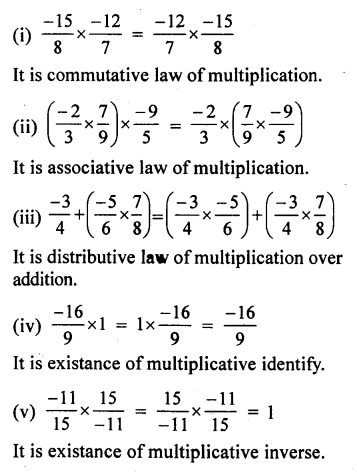Question 9.
Solution:
(i) The product of a rational number and its reciprocal is 1.
(ii) Zero has no reciprocal.
(iii) The numbers 1 and -1 are their own reciprocal.
(iv) Zero is not the reciprocal of any number.
(v) The reciprocal of a, where a≠0, is $$\\ \frac { 1 }{ a }$$
(vi) The reciprocal of $$\\ \frac { 1 }{ a }$$ where a≠0 is a
(vii) The reciprocal of a positive rational number is positive.
(viii) The reciprocal of a negative rational number is negative.

Hope given RS Aggarwal Solutions Class 8 Chapter 1 Rational Numbers Ex 1D are helpful to complete your math homework.

If you have any doubts, please comment below. Learn Insta try to provide online math tutoring for you.